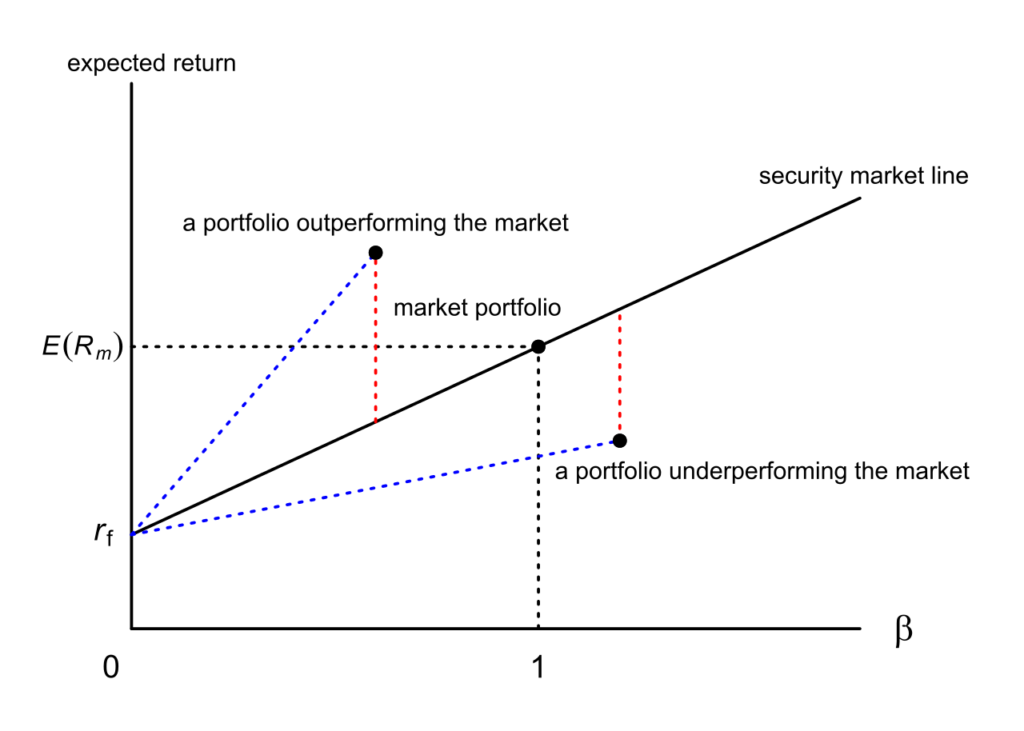# What Is Capital Asset Pricing Model (CAPM)?

## Capital Asset Pricing Model (CAPM) Overview

The Capital Asset Pricing Model, or CAPM, calculates the value of a security based on the expected return relative to the risk investors incur by investing in that security.

To calculate the value of a stock using CAPM, multiply the volatility, known as “beta“, by the additional compensation for incurring risk, known as the “Market Risk Premium”, then add the risk-free rate to that value.

## CAPM Formula

The formula for CAPM is as follows:=expected return=”risk-free” rate of interest=beta (aka volatility – this estimates risk)=expected return of the market

In layman’s terms, the CAPM formula is:

Expected return of the investment = the risk-free rate + the beta (or risk) of the investment * the expected return on the market – the risk free rate (the difference between the two is the market risk premium).

For each additional increment of risk incurred, the expected return should proportionately increase.

If a security is found to have a higher return relative to the additional risk incurred, then the CAPM model suggests that it is a buying opportunity.

Like all valuation models, CAPM has its limitations since some assumptions it uses are idealistic.

For example, Beta coefficients are unpredictable, change over time, only reflect systemic risk rather than total risk.

Despite its shortcomings, this model is very popular for valuing securities.## CAPM FAQs

CAPM stand for “Capital Asset Pricing Model” and is a common valuation method for stocks.
The Capital Asset Pricing Model, or CAPM, calculates the value of a security based on the expected return relative to the risk investors incur by investing in that security.
To calculate the value of a stock using CAPM, multiply the volatility, known as “beta,” by the additional compensation for incurring risk, known as the “Market Risk Premium,” then add the risk-free rate to that value.
Expected return of the investment = the risk-free rate + the beta of the investment times the expected return on the market – the risk free rate which is the market risk premium.

## About the AuthorTrue Tamplin, BSc, CEPF®

True Tamplin is a published author, public speaker, CEO of UpDigital, and founder of Finance Strategists.

True is a Certified Educator in Personal Finance (CEPF®), a member of the Society for Advancing Business Editing and Writing, contributes to his financial education site, Finance Strategists, and has spoken to various financial communities such as the CFA Institute, as well as university students like his Alma mater, Biola University, where he received a bachelor of science in business and data analytics.

To learn more about True, visit his personal website, view his author profile on Amazon, or check out his speaker profile on the CFA Institute website.